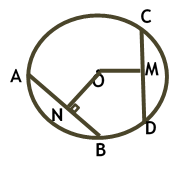Maths / Circles / Equal Chords are Equidistant from the Center of Circle

QUESTION

 In the figure given , if CD = AB = 6 units and ON = 3 units, and OM is perpendicular to CD, then OM + MD is equal toOPTIONS A. 3 units B. 15 units C. 9 units D. 6 units
Right Option : D

EXPLANATION
Explain TypeExplanation Content
Text

As CD = AB = 6 units and ON = 3 units, and OM is perpendicular to CD,

then OM = 3 units as equal chords are equidistant from the centre

As OM is perpendicular so it bisects the chord

MD = 3 units

OM + MD = 3 + 3 = 6 units

TestimonialsPARENT FEEDBACK c/o Om Umang C/o ABHYAS Academy
10th
Abhyas is a complete education Institute. Here extreme care is taken by teacher with the help of regular exam. Extra classes also conducted by the institute, if the student is weak.